# JP2005181097A - Ultrasonic fluid measuring device - Google Patents

## Info

Publication number
JP2005181097A
JP2005181097A JP2003422003A JP2003422003A JP2005181097A JP 2005181097 A JP2005181097 A JP 2005181097A JP 2003422003 A JP2003422003 A JP 2003422003A JP 2003422003 A JP2003422003 A JP 2003422003A JP 2005181097 A JP2005181097 A JP 2005181097A
Authority
JP
Japan
Prior art keywords
time
ultrasonic
fluid
means
measurement
Prior art date
Legal status (The legal status is an assumption and is not a legal conclusion. Google has not performed a legal analysis and makes no representation as to the accuracy of the status listed.)
Withdrawn
Application number
JP2003422003A
Other languages
Japanese (ja)
Inventor
Masanobu Sakai
Shuji Torii

Original Assignee
Nissan Motor Co Ltd

Priority date (The priority date is an assumption and is not a legal conclusion. Google has not performed a legal analysis and makes no representation as to the accuracy of the date listed.)
Filing date
Publication date
Application filed by Nissan Motor Co Ltd, 日産自動車株式会社 filed Critical Nissan Motor Co Ltd
Priority to JP2003422003A priority Critical patent/JP2005181097A/en
Publication of JP2005181097A publication Critical patent/JP2005181097A/en
Withdrawn legal-status Critical Current

• 239000002737 fuel gas Substances 0.000 claims description 20
• 239000000446 fuel Substances 0.000 claims description 18
• 230000000875 corresponding Effects 0.000 claims description 14
• 230000003321 amplification Effects 0.000 claims description 8
• 238000003199 nucleic acid amplification method Methods 0.000 claims description 8
• 230000001902 propagating Effects 0.000 claims 1
• 239000007789 gases Substances 0.000 description 16
• 238000000034 methods Methods 0.000 description 12
• 230000003213 activating Effects 0.000 description 3
• 230000000694 effects Effects 0.000 description 2
• 239000001257 hydrogen Substances 0.000 description 1
• 239000000126 substances Substances 0.000 description 1

## Images

•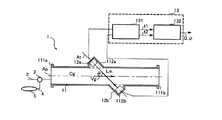•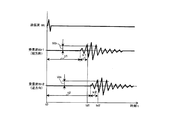•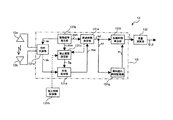•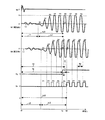•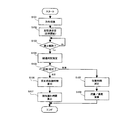•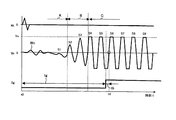•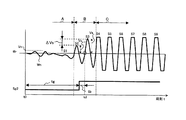•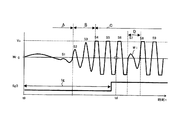••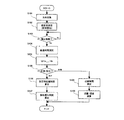•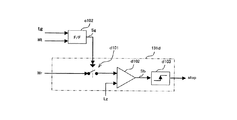•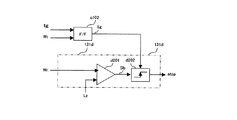•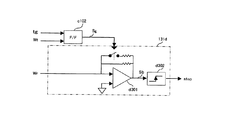•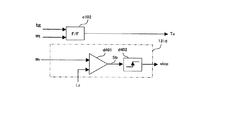•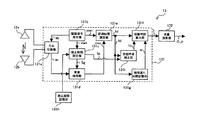•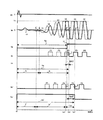•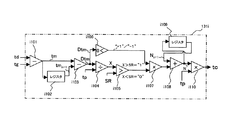•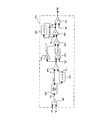•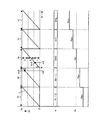•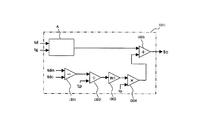•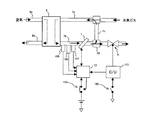•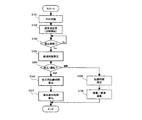•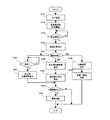## Classifications

• GPHYSICS
• G01MEASURING; TESTING
• G01NINVESTIGATING OR ANALYSING MATERIALS BY DETERMINING THEIR CHEMICAL OR PHYSICAL PROPERTIES
• G01N2291/00Indexing codes associated with group G01N29/00
• G01N2291/02Indexing codes associated with the analysed material
• G01N2291/028Material parameters
• G01N2291/02836Flow rate, liquid level
• GPHYSICS
• G01MEASURING; TESTING
• G01NINVESTIGATING OR ANALYSING MATERIALS BY DETERMINING THEIR CHEMICAL OR PHYSICAL PROPERTIES
• G01N2291/00Indexing codes associated with group G01N29/00
• G01N2291/02Indexing codes associated with the analysed material
• G01N2291/028Material parameters
• G01N2291/02845Humidity, wetness
• GPHYSICS
• G01MEASURING; TESTING
• G01NINVESTIGATING OR ANALYSING MATERIALS BY DETERMINING THEIR CHEMICAL OR PHYSICAL PROPERTIES
• G01N2291/00Indexing codes associated with group G01N29/00
• G01N2291/02Indexing codes associated with the analysed material
• G01N2291/028Material parameters
• G01N2291/02872Pressure
• GPHYSICS
• G01MEASURING; TESTING
• G01NINVESTIGATING OR ANALYSING MATERIALS BY DETERMINING THEIR CHEMICAL OR PHYSICAL PROPERTIES
• G01N2291/00Indexing codes associated with group G01N29/00
• G01N2291/02Indexing codes associated with the analysed material
• G01N2291/028Material parameters
• G01N2291/02881Temperature

## Abstract

In an ultrasonic measurement apparatus, a zero cross point is accurately specified when a test fluid having a large acoustic attenuation is used.
After a ultrasonic wave is emitted from one transducer, a period until a predetermined time elapses is set as a prohibition period, and specification of a zero cross point is prohibited in this prohibition period. The zero cross point is specified from the received waveform Wr after the prohibition period has elapsed. The prohibition period is set with reference to the assumed S / N ratio and excluding the period in which the reception waveform Wr is attenuated due to the influence of the interference wave other than the direct propagation wave. The specific prohibition is due to prohibiting the output of the reception waveform Wr itself during the prohibition period or the input of the reception waveform Wr to identify the zero cross point internally.
[Selection] Figure 4

## Description

The present invention relates to an ultrasonic fluid measuring device, and more particularly to a technique for accurately specifying a zero-crossing point that is a reference for propagation time measurement when acoustic attenuation of a test fluid is large.

Conventionally, a pair of transducers are arranged shifted in the direction of flow, and an ultrasonic wave is emitted from the upstream transducer in the forward direction with respect to the flow, and the first propagation time until this is received by the downstream transducer is And measuring the second propagation time until it is received by the upstream transducer from the downstream transducer and measuring the second propagation time until it is received by the upstream transducer. In addition, a fluid measuring device for detecting the flow rate of a test fluid is known. In this ultrasonic fluid measuring device, when measuring the first and second propagation times, a point of time when the output voltage of the transducer passes a zero level, called a zero cross point, is specified, and from the point of time when the ultrasonic wave is emitted. The time to the zero cross point is measured. In general, the zero-cross point is specified by setting a threshold value related to the output voltage of the transducer in advance and specifying the time point when the actually obtained output voltage reaches this threshold value (patent) Reference 1).
JP 2002-323361 A (paragraph numbers 0033 and 0034)

However, the above ultrasonic fluid measuring device has the following problems when the test fluid has a large acoustic attenuation such as hydrogen gas.
That is, when hydrogen gas or the like is used as the test fluid, the emitted ultrasonic wave is greatly attenuated before reaching the receiving-side transducer. For this reason, while the ultrasonic component of a transducer output becomes small, the noise component superimposed on this becomes relatively large. For this reason, in the above method by comparing the output voltage of the transducer and the threshold value, when a large noise is accidentally input before the original zero cross point and the output voltage reaches the threshold value, In this case, an erroneous zero cross point (which is earlier than the original zero cross point) is specified. On the other hand, in order to avoid this, if a large threshold is set with a margin for the noise component, it may fail to specify the original zero cross point depending on the degree of attenuation of the ultrasonic wave and the size of the noise. Conceivable. If the zero-cross point is incorrectly specified, a large error corresponding to the frequency of the ultrasonic wave is caused in the measurement result such as the flow rate.

The present invention makes it possible to accurately identify the zero-crossing point without being affected by noise components and to obtain an accurate measurement result when a test fluid having a large acoustic attenuation such as hydrogen gas is used. Objective.

The present invention provides an ultrasonic fluid measuring device. An apparatus according to the present invention includes a measurement tube for circulating a test fluid, a first transducer that is installed on an ultrasonic propagation line set across the measurement tube, and emits ultrasonic waves in a forward direction with respect to the flow; A second transducer that is disposed downstream of the first transducer on the ultrasonic wave propagation line and emits ultrasonic waves in a direction opposite to the flow is configured. A time point at which the output of the transducer that has received the ultrasonic wave emitted from the first or second transducer reaches a predetermined level is specified as a reception time point, and from the first or second transducer based on the specified reception time point Each propagation time of the emitted ultrasonic wave to the transducer on the receiving side is measured, and a predetermined calculation regarding the test fluid is performed based on each measured propagation time. Here, after the ultrasonic wave is emitted from the first or second transducer, a period until a predetermined time elapses is set as a prohibited period, and in this prohibited period, specification of the reception time is prohibited.

In the present invention, when specifying the reception time point, this specification is prohibited until a predetermined time elapses after the ultrasonic wave is emitted (that is, the prohibition period). For this reason, even when the attenuation of ultrasonic waves is large and a relatively large noise component is superimposed on the transducer output, the reception time point is erroneously specified by the noise that is accidentally input before the original reception time point. Can be prevented. In addition, by preventing the erroneous specification of the reception time point in this way, it is possible to optimize the predetermined level regarding the specification of the reception time point, and the ultrasonic wave having a certain level in comparison with the predetermined level. Since components can be employed, the reception time point can be accurately and reliably specified.

Embodiments of the present invention will be described below with reference to the drawings.
FIG. 1 shows the configuration of an ultrasonic fluid measurement device (hereinafter referred to as “measurement device”) 1 according to a first embodiment of the present invention. In this embodiment, hydrogen gas is used as the test fluid.
The measuring pipe 11 forms a passage for measuring a flow rate or the like, and is connected to the adjacent pipe 2 via flanges 111a and 111b formed at each end in the axial direction. A direction switching valve 3 is interposed in the pipe 2, and the measurement pipe 11 is configured to be selectively connected to the pipe 2 and the other pipe 4 by the direction switching valve 3. A tank 5 filled with nitrogen gas as a calibration fluid is connected to the other pipe 4, and the nitrogen gas in the tank 5 can be circulated to the measuring pipe 11.

The measuring tube 11 swells in a cylindrical shape at two locations shifted in the tube axis direction, with an axis forming an angle θ between the measuring tube 11 and the tube axis Ap (corresponding to the ultrasonic wave propagation axis At) as the center. The pair of transducer cases 112a and 112b formed in this way include an upstream transducer (corresponding to a “first transducer”) 12a and a downstream transducer (corresponding to a “second transducer”) 12b. Are stored respectively.

These transducers 12 a and 12 b are configured to include a transducer that generates the measurement ultrasonic wave Wt, and are connected to the control unit 13 including a propagation time calculation unit 131 and a flow rate calculation unit 132. The control unit 13 generates a drive signal for emitting the ultrasonic wave Wt to each of the transducers 12a and 12b, and inputs the reception signals Wr1 and Wr2 output from the transducer that has received the emitted ultrasonic wave Wt. . Each transducer 12a, 12b receives a drive signal from the control unit 13 and emits an ultrasonic wave Wt along the ultrasonic propagation line At. The control unit 13 calculates and outputs the flow rate Q and density (corresponding to the concentration) ρ of the hydrogen gas flowing through the measurement tube 11 based on the received reception signals Wr1 and Wr2. The output flow rate Q and the like are displayed on a monitor (not shown).

Here, the measurement principle of the flow rate Q and the density ρ by the measuring device 1 will be described with reference to FIG.
FIG. 2 shows an ultrasonic transmission waveform Wt and reception waveforms Wr1 and Wr2. The received waveform obtained when the ultrasonic wave Wt is emitted in the forward direction with respect to the flow from the upstream transducer 12a is Wr1, and obtained when the ultrasonic wave Wt is emitted in the reverse direction with respect to the flow from the downstream transducer 12b. The received waveform is Wr2. After the ultrasonic wave Wt is emitted, the reception time point td is the time when the reception signal (indicated by voltage) reaches a predetermined level Vth, and the first ultrasonic wave (hereinafter referred to as “first wave”) is received by the receiving transducer. The time from reception until the reception time td is defined as a detection delay time tr, and the time from when the ultrasonic wave Wt is emitted until the leading wave reaches the receiving transducer is defined as a propagation time t. Assuming that the sound velocity and flow velocity of hydrogen gas and the propagation distance of the ultrasonic wave Wt are Cg, Vg, and Lm, the ultrasonic wave Wt is emitted in the forward direction and the ultrasonic wave Wt is emitted in the reverse direction. Between the time td and the time tr, t, the following expressions (1) and (2) are satisfied.

td1 = t1 + tr1
= Lm / (Cg + Vg × cos θ) + tr1 (1)
td2 = t2 + tr2
= Lm / (Cg−Vg × cos θ) + tr2 (2)
When measuring the flow rate Q, the following equation (3) relating to the flow velocity Vg is obtained based on the equations (1) and (2).

Vg = [Lm / (2 × cos θ)] × {1 / (td1-tr1) −1 / (td2-tr2)} (3)
Substituting the flow velocity Vg calculated by the equation (3) into the following equation (4), the flow rate Q is calculated. The cross-sectional area of the measuring tube 11 is A, and the flow velocity distribution correction coefficient in the measuring tube 11 is K.
Q = Vg × A × K (4)
On the other hand, when measuring the density ρ, the sound velocity Cg is calculated by the following equation (5).

Cg = (Lm / 2) × {1 / (td1-tr1) + 1 / (td2-tr2)} (5)
The sound velocity Cg calculated by the equation (5) is substituted into the following equation (6) to calculate the density ρ. The specific heat ratio is γ, the gas constant is R, and the temperature is T.
ρ = γ × {R × T / (22.4 × Cg 2 )} (6)
In the present embodiment, the ultrasonic propagation line At is set in a straight line, and the pair of transducers 12a and 12b is disposed on each side of the measurement tube 11 with the tube axis Ap as a reference. However, according to the present invention, the present invention is not limited to such an arrangement, and both transducers can be arranged only on one side of the measurement tube 11 by setting the ultrasonic propagation line At to bend on the tube wall. .

FIG. 3 shows a configuration of the control unit 13 according to the present embodiment. The configuration of the control unit 13 will be described with reference to FIG. 3 with reference to the time chart shown in FIG.
The direction switching unit 131a selects one transducer (for example, the upstream transducer 12a) for transmission and selects the other downstream transducer 12b for reception.

The drive signal generator 131b generates a drive signal for the transmission transducer. The generated drive signal is input to the transmission transducer via the direction switching unit 131a, and the ultrasonic wave Wt is emitted (time t0). The drive signal generation unit 131b outputs a time measurement start signal start to the prohibition period setting unit 131c and the elapsed time measurement unit 131e simultaneously with the generation of the drive signal.

When the prohibition period setting unit 131c receives the measurement start signal start, the prohibition period setting unit 131c outputs a reception control signal Sg that prohibits specification of the reception time point over a predetermined prohibition period tg to the reception detection unit 131d. The reception control signal Sg is set to Lo level during the prohibition period tg, and is switched to Hi level after the prohibition period tg has elapsed. While the reception control signal Sg is set at the Lo level, the reception detection unit 131d is prohibited or substantially prohibited from specifying the reception time point, and the reception control signal Sg is switched to the Hi level, thereby specifying the reception time point. Allowed. The prohibition period tg is stored in the prohibition period storage unit 131h and read into the prohibition period setting unit 131c.

The reception detection unit 131d receives the reception signal Wr from the transducer selected for reception by the direction switching unit 131a. Here, the reception detection unit 131d suspends specification of the reception time point while converting the reception signal Wr to the rectangular signal Sb until the input reception control signal Sg is switched to the Hi level indicating the cancellation of prohibition. The reception detecting unit 131d specifies the first rising point C ′ of the converted rectangular waveform Sb as the reception time after the reception control signal Sg is switched to the Hi level, and at the specified rising point C ′, the elapsed time A time measurement end signal stop is output to the measurement unit 131e. The rising point C ′ corresponds to the zero cross point according to the present embodiment.

The elapsed time measuring unit 131e operates the timer by inputting the time measurement start signal start, stops the timer by inputting the time measurement end signal stop, and outputs the time measurement result td as the elapsed time to the propagation time calculation unit 131f.
The propagation time calculation unit 131f calculates the propagation time t by the following equation (7) based on the input elapsed time td and the predetermined detection delay time tr. The propagation time calculation unit 131f outputs the calculated propagation time t to the flow rate calculation unit 132. The detection delay time tr is stored in the detection delay time storage unit 131g and read into the propagation time calculation unit 131f. The detection delay time tr is updated according to a calibration process described later.

t = td−tr (7)
The flow rate calculation unit 132 calculates the flow rate Q and density ρ of the hydrogen gas using the equations (3) to (6) based on the input propagation time t.
Next, the operation of the control unit 13 will be described with reference to the flowchart shown in FIG.

When the ultrasonic fluid measuring device 1 is powered on, this routine is started and initial setting is performed. This routine is executed every predetermined time.
In S101, the ultrasonic wave propagation direction is switched.
In S102, an ultrasonic wave is emitted from the transmission transducer, and the elapsed time td is started.

In S103, it is determined whether the prohibition period tg has elapsed and the reception control signal Sg has been switched to the Hi level to allow the specification of the reception time point. This determination is repeated until permission is granted, and if permission is granted, the process proceeds to S104.
In S104, the zero cross point C ′ is specified, and the elapsed time td is measured based on the time td of the specified zero cross point C ′.

In S105, it is determined whether calibration is being performed or measurement is being performed. In this embodiment, switching between calibration and measurement is manually performed by a switch, and this determination is performed by detecting an output signal of the switch. During calibration, the measurement tube 11 is filled with nitrogen gas, and during measurement, hydrogen gas is circulated through the measurement tube 11.
In S106, the propagation time for calibration according to the sound speed of nitrogen gas is calculated by the equation (1). The flow rate Vg of the calibration fluid is detected by installing a simple sensor on the pipe 4. When a single composition gas such as nitrogen gas or hydrogen gas is used as the calibration fluid, the sound velocity Cg is expressed as follows: specific heat ratio is γ, gas constant is R, temperature is T, molar mass is M, It calculates by Formula (8).

Cg = √ (γ × R × T / M) (8)
In S107, the detection delay time tr is calculated by subtracting the calculated propagation time from the measured elapsed time td. In the calibration, an elapsed time td may be obtained by emitting ultrasonic waves a plurality of times and averaging the elapsed times measured for each emission. The calculated detection delay time tr is stored in the detection delay time storage unit 131g. When the calibration is completed, the switch is switched and hydrogen gas flows through the measurement tube 11.

In S108, the propagation time t is calculated by subtracting the detection delay time tr from the measured elapsed time td.
In S109, based on the calculated propagation time t, the flow rate Q and the density ρ of the hydrogen gas are calculated by the equations (3) to (6).
Next, the blocks 131a to 131h constituting the control unit 13 will be described in detail.

First, the prohibition period setting unit 131c will be described.
FIG. 6 shows a case where the periods A and B until the reception waveform Wr becomes steady are adopted as the prohibition period. The reception waveform Wr receives an ultrasonic pressure and gradually increases in amplitude from the leading wave S1 in each cycle according to the operating characteristics of the transducers 12a and 12b, and eventually reaches a maximum amplitude and becomes steady. (Period C). By allowing the specification of the reception time point (that is, the zero cross point) in the period C in which the amplitude of the received waveform Wr is maximum, it is possible to avoid the erroneous zero cross point being specified before the original zero cross point, After the specification of the zero cross point is permitted, the maximum S / N ratio is obtained for the noise component Wn, and the zero cross point can be accurately specified. In the prohibition period, a margin time tb for the zero cross point is provided.

FIG. 7 shows a case where the period A until the amplitude fluctuation amount ΔVs (= Vs n −Vs n−1 ) for each period of the received waveform becomes sufficiently larger than the amplitude Vn of the noise component Wn is adopted as the prohibition period. Show. The leading wave S1 is buried in the noise component Wn and often cannot be distinguished from the noise component Wn. However, for example, by allowing the specification of the zero cross point when the amplitude fluctuation amount ΔVs for each period is twice or more the amplitude Vn of the noise component Wn, the influence of the noise component Wn is suppressed, and the received waveform Wr is reduced. The zero cross point can be identified early before the steady state. According to this method, since the measurement cycle can be shortened, responsiveness to changes in flow rate and the like can be improved. The margin time tb for the zero cross point is provided as described above.

FIG. 8 employs a period D in which the received waveform Wr is partially attenuated by Wi due to interference with a wave other than the ultrasonic wave that has propagated on the ultrasonic wave propagation line At, in addition to any of the above, as the prohibition period. Shows the case. Much of the attenuation of the received waveform Wr is generated when the ultrasonic wave that has deviated from the ultrasonic wave propagation line At is reflected by the tube wall of the measurement tube 11 and enters the receiving transducer. For this reason, it is possible to specify the phase lag time of the reflected propagation wave, that is, the period D, based on the dimensions of the measuring tube 11 and the density of the test fluid. According to this method, it is possible to prevent erroneous specification of the zero cross point due to attenuation of the received waveform Wr. The margin time tb for the zero cross point is provided as described above.

The prohibition period set as described above is stored in the prohibition period storage unit 131h (FIG. 3) and read into the prohibition period setting unit 131c. The prohibition time setting unit 131c sets the reception control signal Sg to the Lo level until the read prohibition period elapses, prohibits the specification of the zero cross point, and after this, the reception control signal Sg is set to Hi. Switch to level and allow zero-cross point identification.

In addition, a plurality of prohibition periods having different lengths may be stored in advance in the prohibition period storage unit 131h, and the prohibition period to be employed may be selected according to the type of the test fluid in measurement. The prohibition period can be selected by providing an adjustment dial on the operation panel or by generating an external signal corresponding to each prohibition period. The external signal may be in any form such as an analog voltage or current, a digital signal, an optical signal, or fluid pressure. In the case of using an external signal, the prohibition period can be selected by remote operation, so that the reception detection unit 131d and the like for processing a weak voltage signal can be installed apart from the flow rate calculation unit 132 and the monitor. . For this reason, when the measuring apparatus 1 is used by being incorporated in a chemical plant or radiation facility, the reception detector 131d and the like are installed at a position close to the transducers 12a and 12b, and noise (mainly electromagnetic) is mixed. Can be avoided and measurement accuracy can be ensured.

FIG. 9 shows a configuration of the prohibition period setting unit 131c when the prohibition period length tg is variable. The prohibition period setting unit 131c includes a shift register c101 and a subtractor c102, and is connected to the elapsed time measurement unit 131e (FIG. 3). The elapsed times td1 and td2 in the forward and reverse directions are input, and the average elapsed time ta (= (td1 + td2) / 2) is calculated for each measurement based on the input propagation times td1 and td2. Store in c101. The subtractor c102 inputs the elapsed time average value tan -1 calculated in the previous measurement from the shift register c101, and subtracts the margin time tb from the input elapsed time average value tan -1, to thereby determine the prohibited period. The length tg (= tan -1− tb) is calculated. The prohibition period setting unit 131c outputs the reception control signal Sg at the Lo level over the set prohibition period. As described above, by providing the function of adjusting the length tg of the prohibition period for each measurement, the zero cross point C ′ is always allowed to be identified before a certain time tb (FIG. 4). For this reason, even if the zero cross point C ′ fluctuates around due to a change in the density (that is, the speed of sound) of the test fluid, the identification can be permitted at an optimal timing. The operation of the control unit 13 when the prohibition period setting unit 131c is used will be described with reference to the flowchart shown in FIG.

In the flowchart shown in FIG. 10, steps that perform the same processing as that shown in FIG. 5 are given the same reference numerals.
This routine is started when the power is turned on, and thereafter is executed every predetermined time. After the start, initial setting is performed, the propagation direction of the ultrasonic wave is switched (S101), and the ultrasonic wave is emitted from the transmission transducer (S102). When the prohibition period has elapsed and the specification of the zero cross point is permitted (S103), the process proceeds to S104, where the zero cross point C ′ is specified and the elapsed time td is measured. In S201, the elapsed times td1 and td2 measured in each of the forward and reverse directions are averaged to calculate an elapsed time average value ta, and a predetermined margin time tb is calculated from the calculated average value ta (= tan -1 ). Subtraction is performed to calculate the length tg of the prohibition period. The elapsed time average value ta is stored in the memory c101 and read in this step in the next measurement. It is determined whether it is during calibration or measurement (S105). At the time of calibration, a calibration propagation time corresponding to the sound velocity of the calibration fluid is calculated (S106), and a detection delay time tr is calculated and stored. To do. At the time of measurement, the detection delay time tr is subtracted from the measured elapsed time td to calculate the propagation time t (S108), and based on the calculated propagation time t, hydrogen is calculated according to equations (3) to (6). The gas flow rate Q and the like are calculated (S109).

Although the prohibition period is not limited to the above, it is preferable to set the time tg at which the specification of the zero cross point is permitted in consideration of the maximum flow rate of the test fluid. For example, it is assumed that the time point indicated by the symbol D in FIG. 4 is a zero cross point specified when the flow rate is zero. Here, when the flow rate increases, the zero cross point A specified for the forward direction is advanced accordingly, while the zero cross point C specified for the reverse direction is delayed. By setting the length tg of the prohibition period so that the specification is permitted before the zero cross point specified in the forward direction at the assumed maximum flow rate, an erroneous zero cross point in a cycle other than the target cycle Can be avoided, and the flow rate and the like can be accurately measured over the entire operating range.

Next, the reception detection unit 131d will be described.
FIG. 11 shows the configuration of the reception detection unit 131d. The reception detection unit 131d includes a switch d101, and the switch d101 forms a gate for the reception signal Wr of the transducer. The reception signal Wr is input to the comparator d102 via the switch d101. The prohibition period setting unit 131c is provided with a flip-flop c102 whose input state is switched according to the drive signal of the transducer and the length tg of the prohibition period, and the switch d101 is an output of the flip-flop d101 (ie, reception control) Actuated by signal Sg). The transducer and the comparator d102 are connected when the switch d101 is turned on when the reception control signal Sg is at the Hi level, and are turned off when the switch d101 is turned off. For this reason, in the prohibition period, the input of the reception signal Wr to the comparator d102 is prevented. The comparator d102 outputs a rectangular waveform Sb whose level transitions at each zero cross point with reference to the zero level of the received waveform Wr (FIG. 4). Since the input of the reception signal Wr is blocked during the prohibition period, the output Sb of the comparator d102 is maintained at zero level until the prohibition period elapses. The output Sb of the comparator d102 is input to the monostable multivibrator d103, and the monostable multivibrator d103 specifies the first rising point C ′ of the rectangular waveform Sb as the zero cross point, and outputs the timing stop signal stop.

In addition, by binarizing the reception signal Wr to the Hi level or the Lo level by the comparator d102, the information can be simplified, the calculation efficiency can be improved, and the cost can be reduced. Further, by setting the binarization reference to the zero level of the reception waveform Wr, the level transition point of the rectangular waveform Sb and the zero cross point of the reception waveform Wr coincide with each other, so that the zero cross point can be easily identified.

FIG. 12 shows the configuration of another reception detection unit 131d. In the reception detection unit 131d, the transducer and the comparator d201 are directly connected without using a gate such as a switch. Therefore, the reception signal Wr is always input to the comparator d201, and the comparator d201 outputs a rectangular waveform Sb with reference to the zero level of the reception waveform Wr. The flop flop c102 of the prohibition period setting unit 131c outputs a reception control signal Sg corresponding to the drive signal of the transducer and the length tg of the prohibition period. The reception control signal Sg is output to the monostable multivibrator d202 together with the output of the comparator d201. Entered. The monostable multivibrator d202 internally generates a timing stop signal stop every time the rectangular signal Sb rises, but its output is prohibited by the reception control signal Sg until the prohibition period elapses. As described above, by always specifying the zero cross point internally and prohibiting the output in the prohibition period, the same effect as allowing the specification of the zero cross point after the prohibition period elapses can be obtained.

FIG. 13 shows a configuration of still another reception detection unit 131d. This reception detection unit 131d is configured to include a variable amplifier d301 so that the amplification factor G of the reception signal Wr can be switched between a first value G1 and a second value G2 larger than this. It is configured. The magnitude of the gain G is switched by the reception control signal Sg output from the flip-flop c102 of the prohibition period setting unit 131c. When the reception control signal Sg is at the Lo level, the magnitude G is set to the first value G1 and at the Hi level. At some point, it is set to the second value G2. The received waveform Wr via the variable amplifier d301 is amplified to the extent that it can be detected by the monostable multivibrator d302 when the second value G2 is used, whereas the monostable multivibrator is used when the first value G1 is used. It attenuates so that it is not reliably detected by d302. In this way, by switching the amplification factor G, the specification of the zero cross point in the prohibition period is disabled, and the same effect as that of prohibiting the specification of the zero cross point can be obtained.

FIG. 14 shows a configuration of still another reception detection unit 131d. In the reception detection unit 131d, the reception signal Wr of the transducer is always input to the comparator d401, and the comparator d401 outputs a rectangular signal Sb based on the zero level of the reception waveform Wr to the monostable multivibrator d402. Output. The monostable multivibrator d402 generates a timing stop signal stop every time the rectangular signal Sb rises, and outputs it to the elapsed time measuring unit 131e. Here, the flip-flop c102 of the prohibition period setting unit 131c generates a timing control signal Te corresponding to the transducer drive signal and the prohibition period length tg, and outputs it to the elapsed time measurement unit 131e. As with the reception control signal Sg, the timing control signal Te is set to the Lo level during the prohibition period, and then set to the Hi level. The elapsed time measuring unit 131e stops the timer by inputting the time stop signal stop and outputs the elapsed time td. In the prohibited period, the timer stop is prohibited by the Lo level time control signal Te. Therefore, the elapsed time measuring unit 131e stops the timer after the timing control signal is switched to the Hi level, and outputs the elapsed time td.

Next, the detection delay time storage unit 131g will be described.
The detection delay time storage unit 131g stores the detection delay time tr calculated at the time of calibration (S106, 107). The stored detection delay time tr is read into the propagation time calculation unit 131f at the time of measurement.
In addition, if only the calibration fluid is filled in the measuring tube 11 at the time of calibration and the flow rate Vg is set to zero, a special sensor for detecting the flow rate Vg of the calibration fluid becomes unnecessary, thereby reducing the cost. Can do.

Further, if the elapsed time td is measured for each of the forward direction and the reverse direction at the time of calibration and the average value of the measured elapsed times td1 and td2 is adopted, this average value is used as the elapsed time when the flow rate Vg is zero. And the term of the flow rate Vg can be eliminated. That is, in this case, the detection delay time tr can be calculated by the following equation (9) without detecting the flow rate Vg of the calibration fluid.

tr = {(td1 + td2) / 2}-(Lm / Cg) (9)
The sound velocity Cg of the calibration fluid has a dependence on temperature, and when it is humidified, the dependence on pressure (that is, humidity) is large. Therefore, by detecting the temperature and pressure of the calibration fluid and substituting the detected temperature T and the molar mass M corrected by the pressure into the equation (8), an accurate calibration propagation time can be extended. Can calculate the detection delay time tr.

When a plurality of test fluids having different sound velocities Cg are targeted and measurement is performed by alternately circulating them, the calibration can be simplified as follows.
First, the sound velocities Cgc and Cgm of the calibration fluid and the test fluid are calculated by the following equation (10) obtained by modifying the equation (6).
Cg = √ {γ × R × T / (22.4 × ρ)} (10)
Next, the propagation time for calibration corresponding to the sound velocity Cgc of the calibration fluid is calculated by the following equations (11) and (12).

t1c = Lm / (Cgc + Vg × cos θ) (11)
t2c = Lm / (Cgc−Vg × cos θ) (12)
Based on the sound velocities Cgc and Cgm and the propagation times t1c and t2c calculated as described above, the detection delay times tr1 and tr2 are calculated by the following equations (13) and (14). The symbol td is the elapsed time obtained for each test fluid at the time of measurement.

tr1 = td1−t1c × Cgc / Cgm (13)
tr2 = td2-t2c × Cgc / Cgm (14)
In this way, by storing the sonic velocity Cgc and the calibration propagation times t1c and t2c obtained by calibration, the sonic velocity Cgm is substituted into the equations (13) and (14) when the test fluid is switched. The detection delay time tr can be easily calculated simply by doing. In addition, when hydrogen gas is used as the test fluid, calibration is completed using nitrogen gas that is easy to handle in a factory or the like, and the measurement plant only requires the sonic velocity Cgm of the hydrogen gas. Since calibration with switching is not necessary and the direction switching valve 3 is not necessary, calibration can be simplified and the safety of the measuring apparatus 1 can be improved. The sound speed Cg can be obtained not only by calculation but also separately.

By the way, as described above, the detection delay time tr corresponds to a time obtained by subtracting the propagation time t from the elapsed time td until the zero cross point (FIG. 4). In other words, the detection delay time tr is the time from the arrival of the leading wave S1 to the receiving transducer until the detection target wave (S6 in this case) arrives, and the zero cross point is specified at the rise of the reception waveform Wr. This corresponds to an integral multiple of the ultrasonic cycle Tp. On the other hand, when the zero-cross point is specified at the fall of the reception waveform Wr, it corresponds to an integral multiple of the ultrasonic half-cycle (= Tp / 2). When the number of periods from the arrival of the leading wave S to the arrival of the detection target wave S6 is known, the detection delay time tr can be set in advance based on this relationship. In the example of FIG. 4, since the number of periods is 5, when the ultrasonic frequency is 40 kHz, the detection delay time tr is 125 μs (= Tp × 5 = 25 μs × 5).

tr = n × Tp (15a)
tr = n × (1/2) × Tp (15b)
Next, another embodiment of the present invention will be described.
FIG. 15 shows the configuration of the control unit 13 in the measuring apparatus according to this embodiment. In the figure, the same reference numerals as in FIG. 3 are assigned to blocks that operate in the same manner as in the previous embodiment. The elements other than the control unit 13 such as the measurement tube 11 and the transducers 12a and 12b are the same as those in the previous embodiment.

The control unit 13 further includes a reception time correction unit 131i. When the reception time correction unit 131i specifies a zero-cross point including an error of one cycle or more due to a later-described return (hereinafter simply referred to as “turn-back”) in the change in the elapsed time td, this error is determined. To compensate. The reception time correction unit 131i receives the elapsed time td from the elapsed time measurement unit 131e and the length tg of the prohibition period from the prohibition period setting unit 131c. Is detected, and the period correction amount tc corresponding to the direction is calculated and output to the propagation time calculation unit 131f. The propagation time calculation unit 131f calculates the propagation time t by the following equation (16) based on the elapsed time td, the detection delay time tr, and the input tc.

t = td− (tr−tc) (16)
Here, the folding that occurs when the elapsed time td changes will be described with reference to the time chart shown in FIG. In the figure, a received waveform Wr shows a case where ultrasonic waves are emitted in the forward direction.
Consider a case where the zero-cross point F in the decreasing direction is specified for the wave S4 in the fourth period of the received waveform Wr. First, in the first measurement, the reception control signal Sg is switched to the Hi level at the point H corresponding to the wave S4 in the fourth period, and the specification of the zero cross point F is permitted. The rectangular waveform Sb changes to the Hi level when permission is given, and then changes to the Lo level at the zero cross point F thereafter. For this reason, the correct zero cross point F can be specified by detecting the first falling point I in the rectangular waveform Sb. In the next measurement, it is assumed that the flow rate Q increases and the phase of the received waveform Wr is advanced as indicated by a dotted line. When the flow rate Q increases, the elapsed time td is usually shortened according to the amount of change. However, if the flow rate Q changes rapidly and the point H at which the reception control signal Sg is switched to the Hi level is later than the zero cross point F ′ of the main body, the zero cross at the point H corresponding to the wave S4 in the fourth period. Although the specification of the point is permitted, the first falling point J of the rectangular waveform Sb corresponds to the next wave S5 of the fifth cycle, and therefore an error of one cycle is measured in the measured elapsed time td ′. Will be included. Such a change in the elapsed time td opposite to the original direction is referred to as folding.

FIG. 17 shows a configuration of the reception time correction unit 131i. The subtractor i101 receives the elapsed time td and the length tg of the prohibition period, and calculates the difference tm (= td−tg). This difference tm is the time from when the reception control signal Sg is switched to the Hi level until the zero cross point is specified (FIG. 16). The difference tm is stored in the shift register i102. The subtraction unit i103 inputs the difference tm and the difference tm n−1 calculated in the previous measurement stored in the shift register i102, and calculates the difference Dtm (= tm−tm n−1 ). To do. Note that a predetermined number n of differences tm calculated up to the previous time is stored in the shift register i102, and an average value (= Σtm / n) or a mode value is output to the subtraction unit i103. You may do it. The divider i104 divides the difference Dtm by the ultrasonic period tp, and converts the difference Dtm into a change rate X of the difference tm with the period tp as a reference. The comparator i105 determines whether or not the rate of change X is greater than a predetermined value SR. When the change rate X is large, the comparator i105 outputs 1 to indicate that aliasing has occurred, and outputs 0 when it is small. The predetermined value SR is determined from the rate of change of the flow rate Q and the measurement execution interval. When the change in the flow rate Q that gives a shift of one period to the received waveform Wr is performed over 1 second and the measurement execution interval is 0.1 second, the change in the flow rate Q is linear with respect to time. If it changes, the difference tm (that is, the elapsed time td) will change at a rate of 0.1 (= 1 × 0.1), and when the change rate X exceeding 0.1 is calculated, Therefore, it can be determined that folding has occurred. The predetermined value SR may be set to a value slightly larger than the assumed maximum rate of change in consideration of the fluctuation due to noise. In parallel with the return detection, the sign determination unit i106 divides the difference Dtm by the absolute value to determine the direction of change in the elapsed time td. The sign determination unit i106 outputs “−” when the elapsed time td becomes shorter, and “+” when the elapsed time becomes longer. A multiplier i107 multiplies the output of the comparator i105 by the output of the sign determination unit i106, and determines whether correction for aliasing is necessary. That is, when the output of the multiplier i 107 is 0, it indicates that correction is not necessary, and when it is −1 or 1, it indicates that correction is necessary. In addition, the code | symbol of -1 or 1 shows the direction of correction | amendment. The adder i108 adds the period correction amount N n-1 used in the previous measurement to the output of the multiplier i107, and calculates the current period correction amount N. The period correction amount N is stored in the shift register i109 and is read into the adding unit i108 in the next measurement. By adding the previous period correction amount N n−1 , it is possible to cope with the case where the aliasing occurs a plurality of times. The multiplier i110 multiplies the period correction amount N by the period tp to calculate a period correction amount tc in units of time. The period correction amount tc is output to the propagation time calculation unit 131f.

Regarding the present embodiment, the rate of change X corresponds to a “second variation rate” when the time when the reception control signal Sg is switched to the Hi level is set as the reference time point.
FIG. 18 shows a configuration of another reception time correction unit 131i. The subtractor i201 receives the elapsed time td and the length tg of the prohibition period, and calculates the difference tm (= td−tg).

Here, the difference tm will be described with reference to FIG.
FIG. 19 is a time chart showing the change of the difference tm with respect to the time ts of the original zero cross point F. The difference tm varies between 0 and an ultrasonic cycle tp according to the time ts. As described above, when the phase of the received waveform Wr is advanced due to an increase in the flow rate Q and the time ts of the original zero cross point F is advanced, the difference tm between the elapsed time td and the length tg of the prohibited period is the time ts. It becomes smaller in proportion to the change. When the time ts changes greatly and the zero cross point F comes before the point H, the zero cross point F ′ cannot be specified for the wave s4, and the zero cross point G of the next wave s5 is specified. (FIG. 16). For this reason, the difference tm switches from 0 to tp at the time tg of the point H and further changes in accordance with the decrease in the time tp. As a result, a sawtooth waveform is drawn with respect to the time ts. It becomes. Here, considering the case where the original zero cross point has transitioned from the point F to the point F ′, the difference tm changes from tm1 to tm2 as the zero cross point F changes (FIG. 16). Thus, at time tg, the difference tm is switched to tp, and a turn-back occurs in which the elapsed time td changes in the opposite direction to the original direction (FIG. 19). Hereinafter, an example in which the original zero cross point transitions from the point F to the point F ′ will be described.

Returning to FIG. 18, the section determiner i202 determines which section in the range of the difference tm 0 to tp belongs to. For example, the range of 0 to tp is equally divided into three sections, and it is determined which of the sections 1 to 3 the difference tm belongs to. Assuming that the original zero crossing point is at the point F ′ in this measurement, the interval K to which the difference tm (= tm2) belongs is 3. The determined section K (= 1 to 3) is stored in the shift register i203. The shift register i203 stores the section K n−1 determined last time or at least one section determined up to the previous time. The subtractor i204 inputs the section K and also inputs the section Kn -1 determined last time from the shift register i203, and calculates the difference between these (= K-Kn -1 ). Since the original zero crossing point is at point F in the previous measurement, the difference tm is tm1 and the interval K n-1 is 1. The average value (= ΣK / n) or the mode value of the section K stored from the shift register i203 may be output to the subtractor i204. The equality comparator i205 determines whether or not the interval difference is 2. When it is 2, 1 is output because folding has occurred, and when it is not 2, 0 is output because folding has not occurred. The number of divisions and the width of the section are not particularly limited, and can be appropriately set according to the speed of change of the flow rate Q, the measurement execution interval, and the like. When a value other than 3 is adopted as the number of divisions, the determination value is “number of divisions−1”. In parallel with determining whether or not there is a return, the sign determination unit i205 determines the direction of the return. That is, the sign determination unit i205 compares the section difference with 0, and when the section difference is larger than 0 (when a turn-up in which the elapsed time td increases), the sign determination unit i205 is 1 and when the section difference is 0 or less. -1 is output to. The multiplier i207 multiplies the output of the equal sign comparator i205 and the output of the sign determination unit i206, and calculates the added value N of the period correction amount. When the addition value N is 0, it is not necessary to correct the aliasing, and when the addition value N is −1 or 1, it indicates that this correction is necessary. The adder i208 adds the period correction amount N n−1 used in the previous measurement to the addition value N, and calculates the current period correction amount N (FIG. 19). The period correction amount N is stored in the shift register i209 and read into the adding unit i208 in the next measurement. The multiplier i210 multiplies the period correction amount N by the period tp to calculate a period correction amount tc in units of time. The period correction amount tc is output to the propagation time calculation unit 131f.

In the present embodiment, the section difference (= K−K n−1 ) corresponds to a “second variation rate” when the reference time is set when the reception control signal Sg is switched to the Hi level.
FIG. 20 shows the configuration of still another reception time correction unit 131i. The reception time correction unit 131i is obtained by adding blocks i301 to i305 to the reception time correction unit A described above. The subtractor i301 inputs the elapsed time tdc obtained at the time of calibration and the elapsed time tdm obtained at the start of measurement, and calculates a difference Dtd (= tdm−tdc). The divider i302 receives the difference Dtd and divides by the ultrasonic cycle tp. The integer converter i303 receives the quotient (= Dtd / tp) and converts it to an integer value obtained by rounding down the decimal places. The multiplier i304 multiplies this integer value by the period tp to calculate an initial correction amount tc0 in units of time. The adder i305 adds the initial correction amount tc0 to the period correction amount tc output from the block A, and outputs this to the propagation time calculation unit 131f as a final period correction amount. With this reception time correction unit 131i, in addition to the compensation of the elapsed time being compensated by the block A, the sound velocity differs greatly between the calibration gas and the test gas, and the ultrasonic wave is detected at the detection delay time tc set by the calibration. Even if an error exceeding one period is included, this can be compensated for and the propagation time t can be accurately measured from the beginning of the measurement. The sign of the initial correction amount tc0 is “−” when the sound velocity of the test gas is larger than the sound velocity of the calibration gas, and “+” when the sound velocity is smaller.

In this embodiment, the quotient (= Dtd / tp) corresponds to the “first variation rate” with respect to the reception time point specified at the time of calibration.
FIG. 21 shows the configuration of the fuel cell system in which the measuring device 1 is installed.
The fuel cell 6 is connected to the fuel gas supply pipe 7a and the fuel gas discharge pipe 7b on the anode side, and is connected to the oxidation gas supply pipe 8a and the oxidation gas discharge pipe 8b on the cathode side. Hydrogen gas is supplied to the fuel cell 6 through a fuel gas supply pipe 7a, and air is supplied through an oxidizing gas supply pipe 8a. The fuel gas supply pipe 7a and the fuel gas discharge pipe 7b are connected by a fuel gas return pipe 7c so that a part of the exhaust gas from the anode is returned to the fuel gas supply pipe 7a. The flow rate of the exhaust gas to be returned is adjusted by a flow rate control valve 9 installed downstream of the branching portion 7d. The opening degree of the flow control valve 9 is controlled by a control unit (hereinafter referred to as “fuel cell C / U”) 101 of the fuel cell 6. A measuring device 1 is interposed upstream of the branching section 7d, and a control unit (hereinafter referred to as “measuring device C / U”) 13 of the measuring device 1 sets the measured flow rate Q and density ρ of the exhaust gas. Output to fuel cell C / U101. The fuel cell C / U 101 calculates the opening degree of the flow control valve 9 based on the input flow rate Q, density ρ, and other information. In addition to the measuring device 1, the fuel gas discharge pipe 7b includes a pressure sensor 105 that detects the pressure in the fuel gas discharge pipe 7b, a humidity sensor 106 that detects the humidity of the exhaust gas, and a temperature sensor that detects the temperature of the exhaust gas. 107 is installed, and these detection signals are output to the measuring apparatus C / U13. The measuring device C / U calculates the flow rate Q and density ρ of the exhaust gas based on the received signals Wr of the transducers 12a and 12b, the input pressure, and the like. The fuel cell C / U 101 is provided with a system activation switch 102, and the measuring device C / U 13 is provided with a switch 135 of the measuring device 1. The latter switch 135 is actuated by a command signal from the measuring device C / U13.

FIG. 22 is a flowchart showing the operation of the measuring apparatus C / U13.
When the system activation switch 102 is turned on, the switch 135 is turned on in conjunction with this, and this routine is started. This routine is executed every predetermined time. In this system, when stopped, the fuel gas supply pipe 7a and the fuel gas discharge pipe 7b are filled with nitrogen gas. When the switch 102 is turned on, calibration is performed by this routine, and the detection delay time tr is updated. When the update is completed, hydrogen gas is supplied to the fuel gas supply pipe 7a, the gas in the pipe is switched to hydrogen gas, and the operation of the fuel cell 6 is started. During operation of the fuel cell 6, the measuring device 1 measures the flow rate Q of the exhaust gas. Note that the switch 102 may be provided on the operation panel, and switching between calibration and measurement may be performed manually.

In S101 to 104, the ultrasonic wave propagation direction is switched (S101) as in the flowchart shown in FIG. 5, and the ultrasonic wave is emitted from the transmission transducer (S102). A determination is made (S103), and when this is permitted, the zero cross point C ′ is specified, and the elapsed time td is measured (S104).
In S301, it is determined whether or not calibration is completed. If the calibration is not completed immediately after the switch 135 is turned on, the process proceeds to S106, the calibration propagation time is calculated in the same manner as in the flowchart shown in FIG. 5 (S106), and the detection delay period tr is calculated. Update (S107). When the calibration is completed and the operation of the fuel cell 6 is started, the process proceeds to S108, the propagation time t is measured (S108), and the flow rate Q and the like are calculated (S109).

In this way, since the reception delay time tr can be calibrated every time the system is started, even if a change over time occurs in the circuit systems such as the transducers 12a and 12b and the measuring device F / C13, Compensate and maintain accuracy.
When starting up the system, the supply of hydrogen gas may be started without waiting for the end of calibration, and calibration may be performed using hydrogen gas. In this case, the sonic velocity Cgc of the calibration gas can be accurately calculated by filling or circulating dry pure hydrogen gas in the fuel gas discharge pipe 7b without humidification during calibration. In this way, when the operation of the fuel cell 6 is started after the calibration is completed, since the inside of the pipe has already been switched to hydrogen gas, the output from the fuel cell 6 can be obtained early. it can.

Further, a failure diagnosis function can be added to the measuring device C / U 13 as follows.
FIG. 23 is a flowchart showing the operation of the measurement apparatus C / U 13 having a failure diagnosis function. This routine is started when the system activation switch 135 is turned on, and is executed every predetermined time. In this routine, leaks occurring in the anode side pipe (including the measurement pipe 11, the fuel gas supply pipe 7a, and the fuel gas discharge pipe 7b) are detected by the failure diagnosis function, and the driver is urged to recognize the leak.

In S101-104, the same process as in the flowchart shown in FIG. 5 is performed, and the elapsed time td is measured.
In S401, the operating state of the system is determined. When starting, the process proceeds to S106, during operation, the process proceeds to S108, and when stopped, the process proceeds to S402.
At the time of stoppage, in S402, the humidification is stopped and the flow rate of hydrogen gas same as that at the time of calibration is set so that the flow in the pipe becomes steady. When it becomes steady, the process proceeds to S403. Whether or not it has become steady can be determined by calculating the flow rate Q or the density ρ and comparing the amount of change for each calculation cycle with a predetermined value. Further, it can be easily determined by monitoring a change in temperature, pressure or humidity in the fuel gas discharge pipe 7b with time, or monitoring a time after the switch 135 is turned off.

In S403, the propagation time t is measured and stored as the stop time propagation time. Thereafter, the switch 135 is turned off, and the measuring device 1 is disconnected from the power source.
At start-up, in S106 and 107, the same processing as the flowchart shown in FIG. 5 is performed, and the calibration propagation time and detection delay time tr are calculated and stored.
In S404, it is determined whether or not there is a difference of a predetermined value or more between the stop time propagation time and the calibration propagation time. If there is a difference, the process proceeds to S405, and if there is no difference, this routine is ended as it is.

In S405, an alarm is activated on the assumption that leakage has occurred.
As described above, according to the present invention, after the ultrasonic wave is emitted, the zero cross point is prohibited until the prohibition period elapses, and then the zero cross point is specified. As a result, it is possible to prevent an erroneous zero cross point from being specified before the original zero cross point.

In addition, since the influence of noise on the specification of the zero cross point can be eliminated in this way, the magnitude of the predetermined level Vth relating to the specification of the zero cross point is made appropriate, and is somewhat large in comparison with this level Vth. Since an ultrasonic component having an amplitude can be employed, the zero cross point can be accurately and reliably specified with a good S / N ratio.

Configuration of a measuring apparatus according to an embodiment of the present invention Working principle of measuring device Control unit configuration Measurement device operation Flow chart of measurement routine First setting example of prohibition period Second setting example of prohibition period Third setting example of prohibited period Configuration of the prohibition period setting section when the prohibition period is set to be variable Flow chart of measurement routine in the case of including the prohibition period setting unit First configuration example of the reception detection unit Second configuration example of the reception detection unit Third configuration example of the reception detection unit Fourth configuration example of the reception detection unit Configuration of control unit with period correction function Operation of the measuring device when turnaround occurs First configuration example of the reception time correction unit Second configuration example of the reception time correction unit Same as above Third configuration example of the reception time correction unit Configuration of a fuel cell system equipped with a measuring device Flow chart of measurement routine when applied to fuel cell system Flow chart of measurement routine when it has a fault diagnosis function

Explanation of symbols

DESCRIPTION OF SYMBOLS 1 ... Ultrasonic fluid measuring device, 11 ... Measuring tube, 12a, 12b ... Transducer, 13 ... Measuring device C / U, 131 ... Propagation time calculation part, 132 ... Flow rate calculating part, 3 ... Direction switching valve, 5 ... Calibration gas tank , 6 ... Fuel cell, 7a ... Fuel gas supply pipe, 7b ... Fuel gas discharge pipe, 7c ... Fuel gas return pipe, 8a ... Oxidation gas supply pipe, 8b ... Oxidation gas discharge pipe, 9 ... Flow control valve, 101 ... Fuel Battery C / U, 105 ... Pressure sensor, 106 ... Humidity sensor, 107 ... Temperature sensor.

## Claims (25)

1. A measuring tube for circulating the test fluid;
A first transducer installed on an ultrasonic propagation line set across the measuring tube and emitting ultrasonic waves in a forward direction with respect to the flow;
A second transducer disposed on the ultrasonic propagation line downstream of the first transducer and emitting ultrasonic waves in a direction opposite to the flow;
Reception time specifying means for specifying, as a reception time, a time when the output of the transducer that has received the ultrasonic wave emitted from the first or second transducer reaches a predetermined level;
Propagation time measuring means for measuring each propagation time of the ultrasonic wave emitted from the first or second transducer to the receiving-side transducer based on the specified reception time point;
Based on each measured propagation time, calculation means for performing a predetermined calculation on the test fluid;
Reception time specification prohibiting means for prohibiting reception time specifying means from specifying the reception time in a prohibition period until a predetermined time elapses after the ultrasonic wave is emitted from the first or second transducer. Constructed ultrasonic fluid measuring device.
2.   The ultrasonic fluid measurement device according to claim 1, wherein the propagation time measurement means subtracts a predetermined detection delay time from an elapsed time from an ultrasonic transmission time to a specified reception time, and measures each propagation time. .
3.   The ultrasonic fluid measurement device according to claim 2, wherein the detection delay time is a length that is an integral multiple of one cycle or half cycle of the output waveform of the transducer.
4. The measurement tube is configured to be able to circulate or fill a calibration fluid whose sound velocity is known instead of the test fluid,
The propagation time measurement means includes a time between a reception time point and a transmission time point specified when the calibration fluid is flowing or filled in the measurement tube, and a calibration propagation time obtained based on the sound velocity of the calibration fluid. The ultrasonic fluid measurement apparatus according to claim 2, comprising: means for calculating the difference between the two and means for storing the calculated difference as a detection delay time.
5.   Propagation time measurement means, means for calculating the ratio of the sound velocity of the calibration fluid and the test fluid, means for multiplying the propagation time according to the sound speed of the calibration fluid, to calculate the propagation time for calibration, The ultrasonic fluid measurement device according to claim 4, further comprising:
6.   6. The method according to claim 4, wherein the propagation time measuring means further comprises means for detecting the temperature of the calibration fluid, and means for correcting the sound velocity of the calibration fluid based on the detected temperature. Sonic fluid measuring device.
7.   The propagation time measuring means includes means for detecting the humidity of the calibration fluid, and means for correcting the sound speed of the calibration fluid based on the detected humidity. Ultrasonic fluid measuring device.
8.   The first fluctuation rate of the reception time point specified at the start of measurement relative to the reception time point specified at the time of calibration is calculated, and when the calculated first fluctuation rate is equal to or greater than a predetermined value, the calculated fluctuation rate is The ultrasonic fluid measuring device according to claim 4, further comprising means for correcting the corresponding detection delay time.
9. Means for determining that the flow in the measurement tube is in a steady state under conditions for obtaining a predetermined flow rate of the test fluid;
Means for detecting fluid leakage from the measurement tube based on the propagation time measured by the propagation time measurement means when the flow in the measurement tube is determined to be in a steady state, and the propagation time for calibration; The ultrasonic fluid measuring device according to claim 4, further comprising:
10.   The ultrasonic fluid measuring device according to claim 1, wherein the predetermined time for determining the prohibition period is a time until the amount of change in the amplitude of the output waveform of the transducer for each period becomes equal to or less than a predetermined value.
11.   The predetermined time for determining the prohibition period is a time until the amount of change in each amplitude period in the output waveform of the transducer becomes larger than the amplitude of the noise superimposed before arrival of the ultrasonic wave. The ultrasonic fluid measuring device according to claim 1.
12.   12. The prohibition period is a period in which a predetermined time zone set in advance that an ultrasonic wave other than the ultrasonic wave propagating on the ultrasonic propagation line interferes with the output waveform of the transducer is excluded. The ultrasonic fluid measuring device according to 1.
13. As the predetermined time, a first time and a second time longer than this are set,
The ultrasonic fluid measurement according to claim 1, further comprising means for switching and setting the predetermined time between the first time and the second time with respect to the reception time point specification prohibiting means. apparatus.
14.   14. The ultrasonic fluid measurement apparatus according to claim 13, wherein the setting means includes means for an operator of the apparatus to manually switch the predetermined time.
15.   Measure the elapsed time from the ultrasonic transmission time to the specified reception time for each measurement, and subtract the predetermined margin time from the elapsed time measured in the previous measurement as the predetermined time for the current measurement. The ultrasonic fluid measurement device according to claim 1, further comprising means for setting time.
16.   The ultrasonic fluid measurement device according to claim 15, wherein the elapsed time is an average of the elapsed time measured for each ultrasonic wave emitted from the first and second transducers.
17.   The predetermined time is a time from an ultrasonic wave transmission time point to a time point specified by a reception time point specifying unit at a maximum flow rate and a predetermined time before a reception time point of an ultrasonic wave emitted in a forward direction. Item 18. The ultrasonic fluid measurement device according to any one of Items 1 to 16.
18.   The ultrasonic fluid measurement device according to any one of claims 1 to 17, wherein the reception time point specification prohibiting unit blocks input of a transducer output to the reception time point specifying unit during the prohibition period.
19.   18. The ultrasonic fluid measurement device according to claim 1, wherein the reception time point specification prohibiting unit prohibits the specification of the reception time point when the transducer output reaches a predetermined level during the prohibition period.
20. As the amplification factor of the transducer output, a first amplification factor and a second amplification factor larger than this are set,
18. The reception time point specification prohibiting unit switches the amplification factor before and after the predetermined time has elapsed, and sets the first amplification factor before the passage and the second amplification factor after the passage. The ultrasonic fluid measuring device according to 1.
21.   21. The reception time specifying means includes means for binarizing the transducer output with reference to the predetermined level, and specifies the reception time based on the binarized result. The ultrasonic fluid measuring device according to 1.
22.   The second fluctuation rate of the specified reception time point with respect to a preset reference time point is calculated, and when the calculated second fluctuation rate is equal to or greater than a predetermined value, the calculated fluctuation time is measured with respect to the propagation time measuring means. The ultrasonic fluid measurement device according to any one of claims 1 to 21, further comprising means for correcting a reception time according to a rate.
23.   The ultrasonic fluid measurement device according to claim 22, further comprising means for calculating an average time of at least one reception time point specified up to the previous measurement and setting the calculated average time as the reference time. .
24.   The ultrasonic fluid measurement apparatus according to any one of claims 1 to 23, wherein the calculation means calculates a flow rate or a concentration of the test fluid based on each measured propagation time.
25.   The ultrasonic fluid measuring device according to any one of claims 1 to 24, wherein the test fluid is a fuel gas flowing through an anode pipe of a fuel cell.
JP2003422003A 2003-12-19 2003-12-19 Ultrasonic fluid measuring device Withdrawn JP2005181097A (en)

## Priority Applications (1)

Application Number Priority Date Filing Date Title
JP2003422003A JP2005181097A (en) 2003-12-19 2003-12-19 Ultrasonic fluid measuring device

## Applications Claiming Priority (1)

Application Number Priority Date Filing Date Title
JP2003422003A JP2005181097A (en) 2003-12-19 2003-12-19 Ultrasonic fluid measuring device

## Publications (1)

Publication Number Publication Date
JP2005181097A true JP2005181097A (en) 2005-07-07

# Family

## Family Applications (1)

Application Number Title Priority Date Filing Date
JP2003422003A Withdrawn JP2005181097A (en) 2003-12-19 2003-12-19 Ultrasonic fluid measuring device

## Country Status (1)

JP (1) JP2005181097A (en)

## Cited By (2)

* Cited by examiner, † Cited by third party
Publication number Priority date Publication date Assignee Title
WO2008149868A1 (en) * 2007-05-31 2008-12-11 Teijin Pharma Limited Ultrasonic gas concentration measuring method and device using the same
CN103874920A (en) * 2011-10-06 2014-06-18 韦斯全球有限公司 Exterior wall-coupling type ultrasound system for measuring density and method for same

## Cited By (5)

* Cited by examiner, † Cited by third party
Publication number Priority date Publication date Assignee Title
WO2008149868A1 (en) * 2007-05-31 2008-12-11 Teijin Pharma Limited Ultrasonic gas concentration measuring method and device using the same
JP4988839B2 (en) * 2007-05-31 2012-08-01 帝人ファーマ株式会社 Ultrasonic gas concentration measuring method and apparatus using the same
US8746037B2 (en) 2007-05-31 2014-06-10 Teijin Pharma Limited Ultrasonic apparatus and method for measuring the concentration of gas
CN103874920A (en) * 2011-10-06 2014-06-18 韦斯全球有限公司 Exterior wall-coupling type ultrasound system for measuring density and method for same
JP2014528582A (en) * 2011-10-06 2014-10-27 ウェス グローバル インコーポレーテッドＷｅｓｓ Ｇｌｏｂａｌ， Ｉｎｃ． Clamp-on type ultrasonic concentration measurement system and method

## Similar Documents

Publication Publication Date Title
EP0598720B1 (en) Nonintrusive flow sensing system
US6415660B1 (en) Method and apparatus for the highly accurate determination of the filling level of a product in a container
US9696195B2 (en) Ultrasonic flow switch
US8330942B2 (en) Methods and instruments for estimating target motion
US4459667A (en) Guidance method and system for an automotive vehicle
EP1719980B1 (en) Ultrasonic flowmeter and ultrasonic flow rate measurement method
DE19511234C2 (en) Device and method for water level measurement
US4578997A (en) Time-shaped AGC for ultrasonic liquid level meter of the echo-ranging type
US6158288A (en) Ultrasonic system for measuring flow rate, fluid velocity, and pipe diameter based upon time periods
CN101573596B (en) Method for determining and monitoring the filling level of a medium in a container according to a propagation time measurement method
US5546812A (en) Method and device for determining characteristics of the flow of a medium
JP2918126B2 (en) Fluid level measurement method and device
EP0566708B1 (en) Measurement system
US4805453A (en) Tank sonic gauging system and methods
JP3148737B2 (en) Method of measuring filling level of filling in container by radar
RU2264602C1 (en) Ultrasound method for measuring flow of liquid and/or gaseous substances and device for realization of said method
JP3716274B2 (en) Ultrasonic flow meter and ultrasonic flow measurement method
US7103500B2 (en) Apparatus and method for distance measurement with controlled modulation of emitted pulses
JP2008145275A (en) Flow rate measurement device and gas supply system using same
US6089104A (en) Ultrasonic flow meter using transit time across tube chords for determining the flow rates
JP5402620B2 (en) Flow measuring device
US5939634A (en) Liquid measuring system and methods
US20030136193A1 (en) Ultrasonic apparatus and method for measuring the concentration and flow rate of gas
JP5122453B2 (en) Flow velocity distribution measuring device and ultrasonic flow meter
US5736649A (en) Vortex flowmeter

## Legal Events

Date Code Title Description
A621 Written request for application examination

Free format text: JAPANESE INTERMEDIATE CODE: A621

Effective date: 20061025

RD03 Notification of appointment of power of attorney

Effective date: 20080319

Free format text: JAPANESE INTERMEDIATE CODE: A7423

RD04 Notification of resignation of power of attorney

Free format text: JAPANESE INTERMEDIATE CODE: A7424

Effective date: 20080331

A761 Written withdrawal of application

Free format text: JAPANESE INTERMEDIATE CODE: A761

Effective date: 20090908

A977 Report on retrieval

Free format text: JAPANESE INTERMEDIATE CODE: A971007

Effective date: 20091015